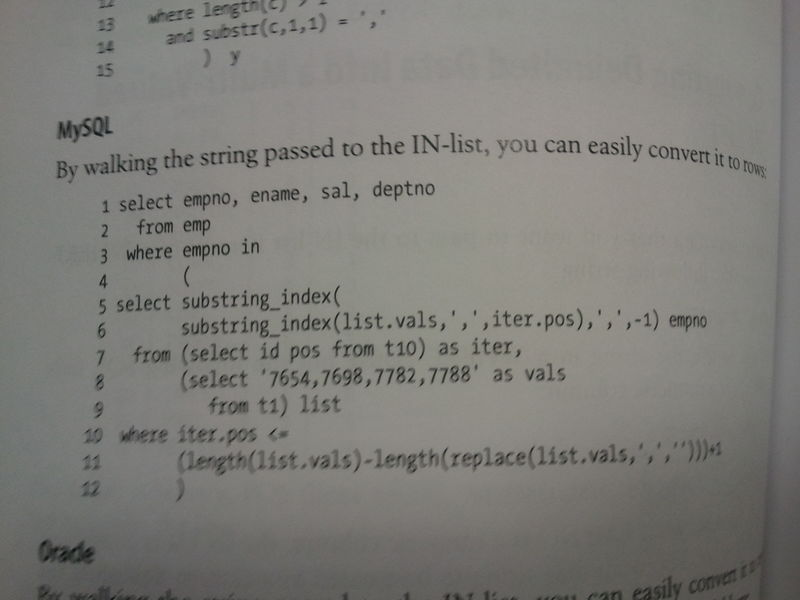## MySQL splitting a comma delimited string into a column of rows

If you want to split a field of your database by commas or some delimiter into a column of rows or a list using pure MySQL here is how you can do it.

I pulled this from the MySQL cookbook and then modified it to not rely on the pivot table t10.

Original Example from MySQL cookbook:SELECT empno, ename, sal, depthno
FROM emp
WHERE empo IN(
SELECT SUBSTRING_INDEX(
SUBSTRING_INDEX(list.vals,',',iter.pos),',',-1
) empno
FROM (SELECT id pos from t10) as iter,
(SELECT '7654,7698,7782,7788' as vals from t1) list
WHERE iter.pos <= (length(list.vals)-length(replace(list.vals,',','')))+1
)

t10 is a table with the column id which has the numbers 1 through 10 listed on each column. This is what the MySQL cookbook calls a pivot table which it a table off of which the MySQL statement iterates. But what if we don't want to use a pivot table. Can we generate a pivot table on the fly. The answer is yes by generating a list of binary numbers using a cross product, convert those to numbers, sort them and eliminate duplicates. If that didn't make sense that is ok, we provide a link bellow that covers it in more detail. For now, just cut and paste the code bellow.

I replaced t10 with the algorithm that generates a list of numbers:

SELECT
f.date FROM
(
SELECT
SUBSTRING_INDEX( SUBSTRING_INDEX(list.vals,',',iter.pos), ',',-1 ) date,
iter.pos,
list.vals,
((length(list.vals)-length(replace(list.vals,',','')))+1) len
FROM
(
(
SELECT
id pos
FROM
(
SELECT
list.id+1 as id
FROM
(
SELECT
(TWO_1.id + TWO_2.id + TWO_4.id + TWO_8.id) id
FROM
(
SELECT
0 id
UNION
ALL
SELECT
1 id
)
TWO_1 CROSS JOIN
(
SELECT
0 id
UNION
ALL
SELECT
2 id
)
TWO_2 CROSS JOIN
(
SELECT
0 id
UNION
ALL
SELECT
4 id
)
TWO_4 CROSS JOIN (SELECT 0 id UNION ALL SELECT 8 id) TWO_8
)
as list
)
as t10
)
as iter CROSS JOIN (SELECT '7654,7698,7782,7788' as vals) list
)
)
as f
WHERE f.pos <= f.len

This will do only 16 comma delimited units. If you want to do more you have to extend the algorithm in t10. You can learn to do this at the following link: